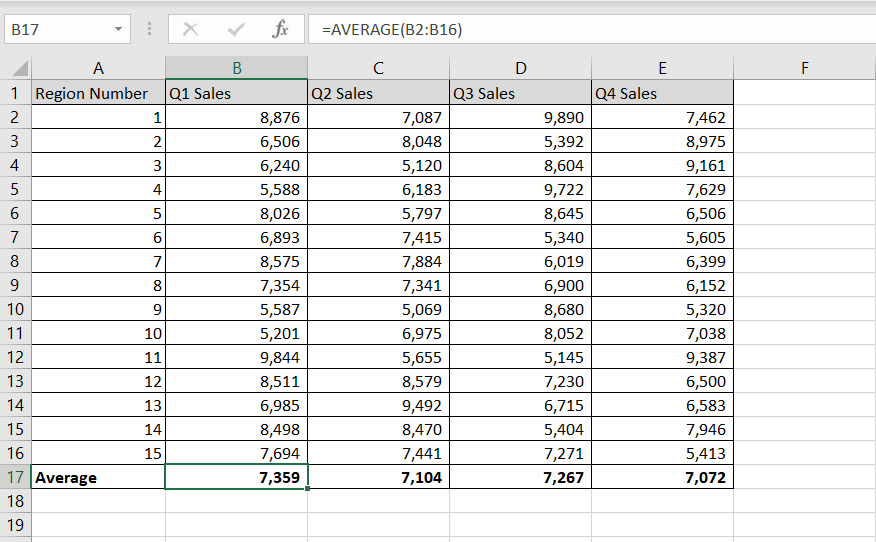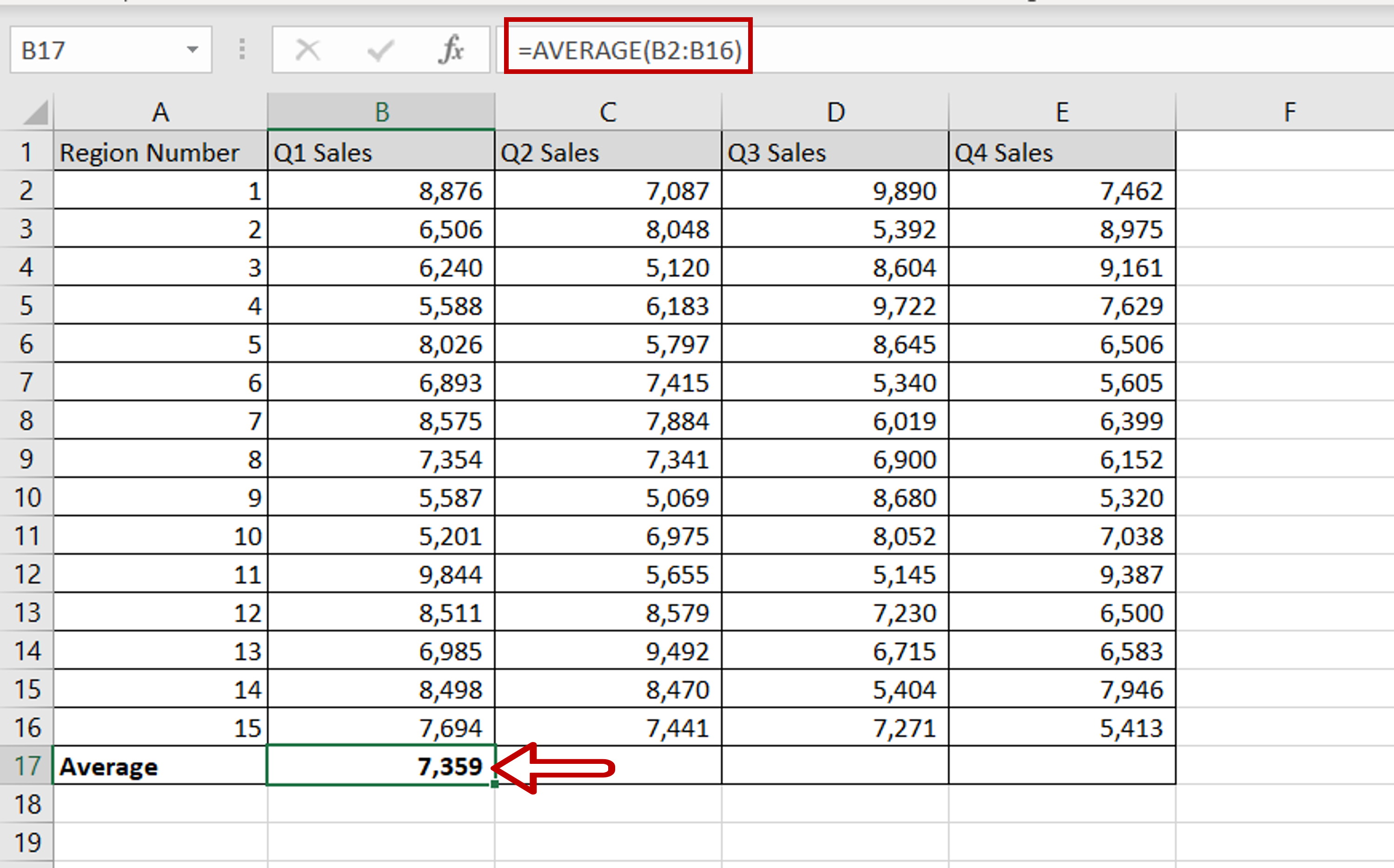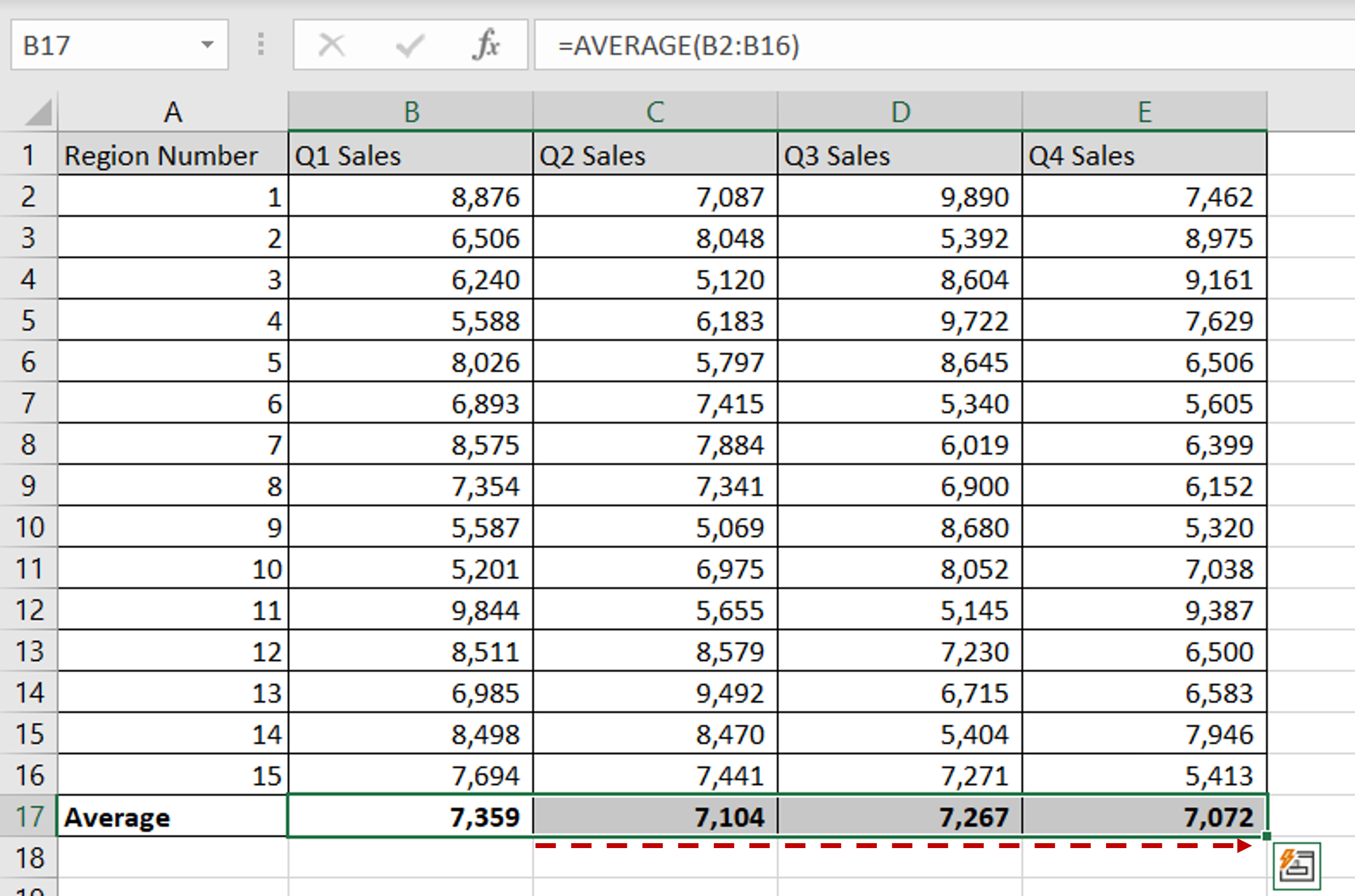# How to average cells in Excel

You can watch a video tutorial here.Excel is widely used for calculations due to the several arithmetic operators and functions that it has. Instead of typing in numbers for calculations, you can use the cell references i.e. the address (Column header & Row number) of the cell that contains the number in the calculation. By doing so, every time the number changes, the calculation or formula is updated automatically. A common operation using numbers is finding the mean or average of a set of numbers. You may have the sales for several regions and you need to find the average across all regions. In Excel, you can do so using the AVERAGE() function.

1. AVERAGE() function: this finds the arithmetic mean of a set of numbers
a. Syntax: AVERAGE(range of numbers)
i. range of numbers: if there is only a single number, then it cannot return the average

### Step 1 – Use the AVERAGE() function– Select the cell in which you want the result to appear
– Type the formula using the cell references:
= AVERAGE (Q1 Sales)
– Press Enter

### Step 2 – Copy the formula– Using the fill handle from the first cell, drag the formula to the remaining cells
OR
a) Select the cell with the formula and press Ctrl+C or choose Copy from the context menu (right-click)
b) Select the rest of the cells in the column and press Ctrl+V or choose Paste from the context menu (right-click